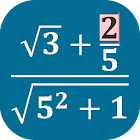Advanced Calculator - Problem Solver

All Android applications categories

All Android games categories# Advanced Calculator - Problem Solver

by: 44 8.8

8.8 Users
rating

## Screenshots

Description

Intuitive input and editing
Saving expressions
On editor, you can use select, copy, cut, paste for expressions
Pinch-To-Zoom
Copy answer
Showing result as decimal or fraction
Undo and redo
Choosing font

SUPPORTED FUNCTIONS
Functions Graphic.
Calculation of mixed, improper fraction and recurring decimal (repeating decimal, periodic numbers).
Periodic number to fraction
Fraction to decimal, Decimal to fraction
Operations with matrices, vectors and complex numbers.
Trigonometric functions: sin, cos, tan, ctan.
The calculation of trigonometric functions in degrees and radians. Use symbol ° for degrees, symbol ' for minute, symbol '' for second.
Inverse trigonometric functions: asin, acos, atan, actan
Secant (sec), Cosecant (csc).
Logarithms: ln, lg, log
Ln: natural logarithm.
Lg: common logarithm.
Constants: π, e
Hyperbolic functions: sh, ch, th, cth
The square root √, the root of n-th degree ⁿ√, Module a , Signum (sign), exponentiation aⁿ.
Combination, Arrangement, Factorial (!)
The sum and product elements of the sequence: Σ, П
Brackets: ( ) [ ] { }
Base conversion of numbers and operations with different base (binary, ternary, quintal, octal, hexadecimal, decimal, base n).
Calculations of limit, definite integral.
Percent (%)
Least (Lowest) Common Multiple (LCM) for fraction and integer numbers
Greatest Common Divisor (GCD) for fraction and integer numbers
Matrices determinant, rang, inverse, addition, subtraction, multiplication, division
Complex numbers addition, subtraction, multiplication, division

from 44 reviews

"Great"

8.8